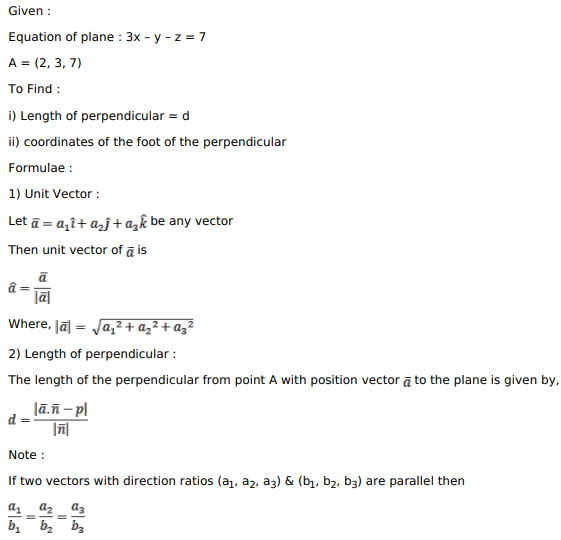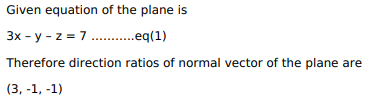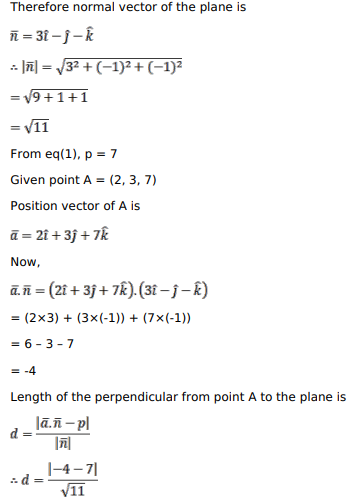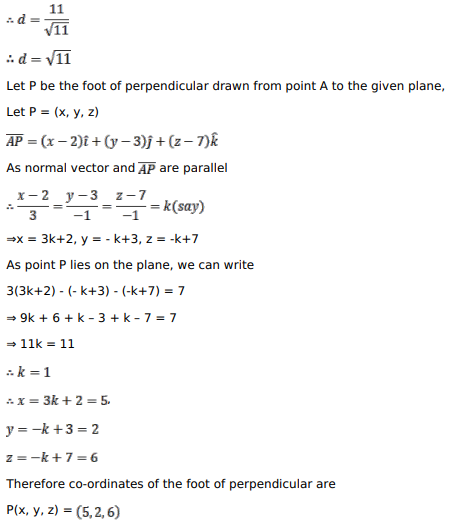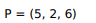# Find the length and the foot of perpendicular drawn from the pointQuestion:

Find the length and the foot of perpendicular drawn from the point $(2,3,7)$ to the plane $3 x-y-z=7$.

Solution: